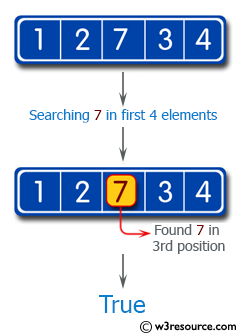﻿ Swift Basic Programming Exercise: check if one of the first 4 elements in a given array of integers is a 7 - w3resource# Swift Basic Programming Exercise: Check if one of the first 4 elements in a given array of integers is a 7

## Swift Basic Programming: Exercise-23 with Solution

Write a Swift program to check if one of the first 4 elements in a given array of integers is a 7. The length of the array may be less than 4.

Pictorial Presentation:Sample Solution:

Swift Code:

``````func arrayFront9(_ input: [Int]) -> Bool {
guard input.count > 3 else {
return false
}

if input.prefix(4).contains(7) {
return true
}
return false
}
print(arrayFront9([1, 2, 7, 3, 4]))
print(arrayFront9([1, 2, 3, 4, 7]))
print(arrayFront9([1, 2, 7]))
```
```

Sample Output:

```true
false
false
```

Swift Programming Code Editor:

Improve this sample solution and post your code through Disqus

What is the difficulty level of this exercise?

﻿